### IMO Shortlist 2010 problem A1

Kvaliteta:
Avg: 5,0
Težina:
Avg: 6,0
Find all function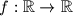$f:\mathbb{R}\rightarrow\mathbb{R}$ such that for all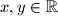$x,y\in\mathbb{R}$ the following equality holds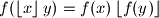where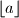$\left\lfloor a\right\rfloor$ is greatest integer not greater than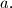$a.$

Proposed by Pierre Bornsztein, France
Izvor: Međunarodna matematička olimpijada, shortlist 2010